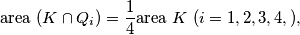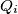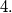### IMO Shortlist 1982 problem 2

Kvaliteta:
Avg: 0.0
Težina:
Avg: 0.0
Let$K$ be a convex polygon in the plane and suppose that$K$ is positioned in the coordinate system in such a way thatwhere the$Q_i$ denote the quadrants of the plane. Prove that if$K$ contains no nonzero lattice point, then the area of$K$ is less than$4.$
Source: Međunarodna matematička olimpijada, shortlist 1982